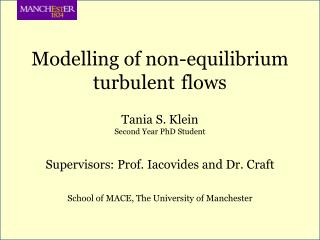DownloadDownload PresentationModelling of non-equilibrium turbulent flows

# Modelling of non-equilibrium turbulent flows

Download Presentation## Modelling of non-equilibrium turbulent flows

- - - - - - - - - - - - - - - - - - - - - - - - - - - E N D - - - - - - - - - - - - - - - - - - - - - - - - - - -
##### Presentation Transcript

1. Modelling of non-equilibrium turbulent flows Tania S. Klein Second Year PhD Student Supervisors: Prof. Iacovides and Dr. Craft School of MACE, The University of Manchester

2. Outline of Presentation • Introduction • Test Cases • Turbulence Models • Results • Conclusions

3. Introduction Non-equilibrium flows: those subjected to rapid changes Sudden contraction, sudden expansion Imposed pressure gradients They are commonly found in the industry: Valves, pumps, heat exchangers, curve surfaces Objective of this work: Test different turbulence models for several cases in order to evaluate their performance.

4. Test Cases • Fully Developed Channel Flow • Homogeneous Constant Shear Flow • Zero Pressure Gradient Boundary Layer • Adverse Pressure Gradient Boundary Layer • Favourable Pressure Gradient Boundary Layer • Contraction/Expansion Flows

5. Fully Developed Channel Flow • One of the simplest flows: • 2D • DP=cte • U=U(y) Simulated Cases ERCOFTAC database Kawamura Lab

6. Homogeneous Constant Shear Flow S=dU/dy=cte  U = U(y) not wall-bounded unsteady

7. Zero Pressure Gradient Boundary Layer Simulated Cases • Still a simple flow: • 2D • DP=0 • U=U(x,y)

8. Adverse Pressure Gradient Boundary Layer • Non-equilibrium flow: • 2D • DP > 0 • U=U(x,y) • dU/dx < 0 S&J M&P

9. Favourable Pressure Gradient Boundary Layer • Non-equilibrium flow: • 2D • DP < 0 • U=U(x,y) • dU/dx > 0 • reaches a self-similar prolife

10. Contraction/Expansion Flows • Non-equilibrium flow: • 3D • dV/dy = cte • dW/dz = -cte a= 0 a= /2

11. Turbulence Models *Run with the wall function of Chieng and Launder (1980)

12. Results Fully Developed Channel Flow General Conclusions • All models predicted the log law reasonably well. • All models predicted the shear Reynolds Stress reasonably well. • The HJ and TC models best predicted the normal Reynolds stresses.

13. Results Fully Developed Channel Flow Re = 6500

14. Results Fully Developed Channel Flow Re = 6500

15. Results Fully Developed Channel Flow Re = 41441

16. Results Homogeneous Constant Shear Flow General Conclusions • Difficult prediction • Overall, the SG and the KS model performed best • The extreme shear values are more difficult to predict. S=20√2 ; S0+=1.68 S=10 ; S0+=16.76

17. Results Homogeneous Constant Shear Flow S=20√2 S0+=1.68

18. Results Homogeneous Constant Shear Flow S=20√2 S0+=30.75

19. Results Zero Pressure Gradient BL General Conclusions • The tested turbulence models have shown to be sensitive to the inlet conditions, implying bad predictions at low Req values. • The normal Reynolds stresses were better predicted by the RST models, as expected. • One can notice the importance of LRN models for the near wall region predictions.

20. Results Zero Pressure Gradient BL

21. Results Zero Pressure Gradient BL

22. Results Adverse Pressure Gradient BL • The BL parameters (Cf, d, d*, q and H) were reasonably well predicted by all turbulence models. • The U and uv profiles were captured by all turbulence models up to station T5 in the S&J case. The same has not occurred for the M&P cases. • The RST models best predicted the normal Reynolds stresses, however the best model varies from case to case; station to station… General Conclusions

26. Results Favourable Pressure Gradient BL General Conclusions • The turbulence model which overall better predicted these flows was the KS model, although it failed to predict the Reynolds stresses. • The KS and LS models are the only ones expected to correctly predict the laminarization process, since they possess a term which accounts for the second derivative of the mean velocities. • The RST models best predicted the normal Reynolds stresses, specially the TC and HJ models.

27. Results Favourable Pressure Gradient BL K=1.5x10-6

28. Results Favourable Pressure Gradient BL K=1.5x10-6

29. Results Favourable Pressure Gradient BL K=2.5x10-6

30. Results Favourable Pressure Gradient BL K=2.5x10-6

31. Results Contraction/Expansion Flows General Conclusions • No turbulence model was able to correctly predict the interruption of the applied strains. • Overall, the GL and the TC models provided the best predictions. • The eddy viscosity formulations clearly failed to predict these flows.

32. Results Contraction/Expansion Flows T&R

33. Results Contraction/Expansion Flows G&M - a = /2

34. Conclusions • The Channel flow, which is the simplest flow, was reasonably well predicted by all turbulence models as well as the ZPGBL cases at high Req values. • The two not wall-bounded cases – HCS flow and C/E flows – were the most difficult to predict and the RST models performed better, showing the importance of calculating the Reynolds stresses through transport equations. • The APGBL cases could not be well predicted by any model at high DP, however the FM model could match the U profile. • The FPGBL cases were better predicted by the KS model which evidenced the importance of a velocity second derivative term to predict laminarization.

35. Thank you